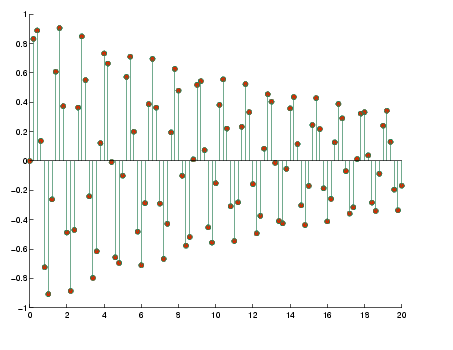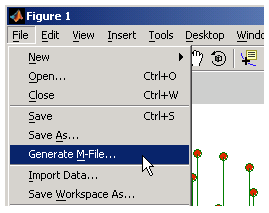GraphicsExample -- Generating M-Code to Reproduce a Graph

Suppose you have created the following graph.

• ```t = 0:.2:20;
alpha =.055;
stem(t,exp(-alpha*t).*sin(5*t))
```

You then use the Property Editor to modify the graph to look like the following picture.You can generate code to reproduce this graph by selecting Generate M-File from the Figure menu. MATLAB creates a function that recreates the graph and opens the generated M-File in the editor.

This feature is particularly useful for capturing property settings and other modifications made using the plot tools GUI.Data Arguments

You must supply the data arguments `t` and `exp(-alpha*t).*sin(5*t)` to the function. Generated functions do not store the data necessary to recreate the graph.

Limitations

Attempting to generate code for graphs containing a large number of graphics objects (e.g., greater than 20 plotted lines) might be impractical.Example -- Specifying a Data Source Editing Plots© 1994-2005 The MathWorks, Inc.